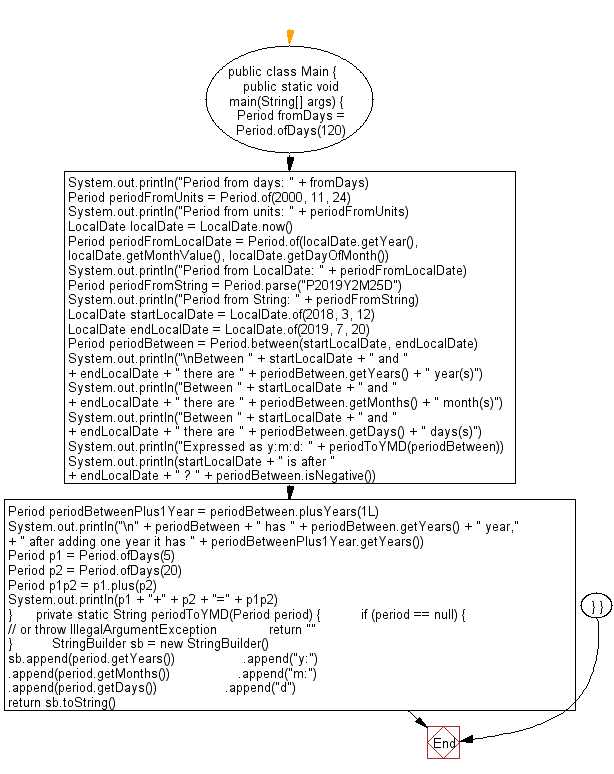﻿ Java exercises: Define a period of time using date-based values and a duration of time using time-based values - w3resource# Java DateTime, Calendar Exercises: Define a period of time using date-based values and a duration of time using time-based values

## Java DateTime, Calendar: Exercise-42 with Solution

Write a Java program to define a period of time using date-based values (Period) and a duration of time using time-based values (Duration).

Sample Solution:

Java Code:

``````//MIT License: https://bit.ly/35gZLa3
import java.time.LocalDate;
import java.time.Period;

public class Main {

public static void main(String[] args) {

Period fromDays = Period.ofDays(120);
System.out.println("Period from days: " + fromDays);

Period periodFromUnits = Period.of(2000, 11, 24);
System.out.println("Period from units: " + periodFromUnits);

LocalDate localDate = LocalDate.now();
Period periodFromLocalDate = Period.of(localDate.getYear(),
localDate.getMonthValue(), localDate.getDayOfMonth());
System.out.println("Period from LocalDate: " + periodFromLocalDate);

Period periodFromString = Period.parse("P2019Y2M25D");
System.out.println("Period from String: " + periodFromString);

LocalDate startLocalDate = LocalDate.of(2018, 3, 12);
LocalDate endLocalDate = LocalDate.of(2019, 7, 20);
Period periodBetween = Period.between(startLocalDate, endLocalDate);
System.out.println("\nBetween " + startLocalDate + " and "
+ endLocalDate + " there are " + periodBetween.getYears() + " year(s)");
System.out.println("Between " + startLocalDate + " and "
+ endLocalDate + " there are " + periodBetween.getMonths() + " month(s)");
System.out.println("Between " + startLocalDate + " and "
+ endLocalDate + " there are " + periodBetween.getDays() + " days(s)");

System.out.println("Expressed as y:m:d: " + periodToYMD(periodBetween));

System.out.println(startLocalDate + " is after "
+ endLocalDate + " ? " + periodBetween.isNegative());

Period periodBetweenPlus1Year = periodBetween.plusYears(1L);
System.out.println("\n" + periodBetween + " has " + periodBetween.getYears() + " year,"
+ " after adding one year it has " + periodBetweenPlus1Year.getYears());

Period p1 = Period.ofDays(5);
Period p2 = Period.ofDays(20);
Period p1p2 = p1.plus(p2);
System.out.println(p1 + "+" + p2 + "=" + p1p2);
}

private static String periodToYMD(Period period) {

if (period == null) {
// or throw IllegalArgumentException
return "";
}

StringBuilder sb = new StringBuilder();
sb.append(period.getYears())
.append("y:")
.append(period.getMonths())
.append("m:")
.append(period.getDays())
.append("d");

return sb.toString();
}
}
```
```

Sample Output:

```Period from days: P120D
Period from units: P2000Y11M24D
Period from LocalDate: P2019Y11M16D
Period from String: P2019Y2M25D

Between 2018-03-12 and 2019-07-20 there are 1 year(s)
Between 2018-03-12 and 2019-07-20 there are 4 month(s)
Between 2018-03-12 and 2019-07-20 there are 8 days(s)
Expressed as y:m:d: 1y:4m:8d
2018-03-12 is after 2019-07-20 ? false

P1Y4M8D has 1 year, after adding one year it has 2
P5D+P20D=P25D
```

N.B.: The value may be changed accroding to your system date and time.

Flowchart:Java Code Editor:

Improve this sample solution and post your code through Disqus

What is the difficulty level of this exercise?

﻿

## Java: Tips of the Day

Directory Content:

Java allows you to get the names of all subdirectories and files in a folder as an array, which can then be sequentially viewed.

```import java.io.*;

public class ListContents {
public static void main(String[] args) {
File file = new File("//home//user//Documents/");
String[] files = file.list();

System.out.println("Listing contents of " + file.getPath());
for(int i=0 ; i < files.length ; i++)
{
System.out.println(files[i]);
}
}
}
```